# 机器学习算法一览（附python和R代码）

2016-04-19 大数据文摘

—— 埃里克 施密特（谷歌首席执行官）

# ◆ ◆ ◆

## 常见的机器学习算法

`1.线性回归 (Linear Regression) 2.逻辑回归 (Logistic Regression) 3.决策树 (Decision Tree) 4.支持向量机（SVM） 5.朴素贝叶斯 (Naive Bayes) 6.K邻近算法（KNN） 7.K-均值算法（K-means） 8.随机森林 (Random Forest) 9.降低维度算法（Dimensionality Reduction Algorithms） 10.Gradient Boost和Adaboost算法`

### 1.线性回归 (Linear Regression)

• Y- 因变量
• a- 斜率
• X- 自变量
• b- 截距

a和b可以通过最小化因变量误差的平方和得到（最小二乘法）。Python 代码

1. `#Import Library`
2. `#Import other necessary libraries like pandas, numpy...`
3. `from sklearn import linear_model`
4. `#Load Train and Test datasets`
5. `#Identify feature and response variable(s) and values must be numeric and numpy arrays`
6. `x_train=input_variables_values_training_datasets`
7. `y_train=target_variables_values_training_datasets`
8. `x_test=input_variables_values_test_datasets`
9. `# Create linear regression object`
10. `linear = linear_model.LinearRegression()`
11. `# Train the model using the training sets and check score`
12. `linear.fit(x_train, y_train)`
13. `linear.score(x_train, y_train)`
14. `#Equation coefficient and Intercept`
15. `print('Coefficient: \n', linear.coef_)`
16. `print('Intercept: \n', linear.intercept_)`
17. `#Predict Output`
18. `predicted= linear.predict(x_test)`

R 代码

1. `#Load Train and Test datasets`
2. `#Identify feature and response variable(s) and values must be numeric and numpy arrays`
3. `x_train <- input_variables_values_training_datasets`
4. `y_train <- target_variables_values_training_datasets`
5. `x_test <- input_variables_values_test_datasets`
6. `x <- cbind(x_train,y_train)`
7. `# Train the model using the training sets and check score`
8. `linear <- lm(y_train ~ ., data = x)`
9. `summary(linear)`
10. `#Predict Output`
11. `predicted= predict(linear,x_test) `

### 2.逻辑回归

`odds= p/ (1-p) = probability of event occurrence / probability of not event occurrence ln(odds) = ln(p/(1-p)) logit(p) = ln(p/(1-p)) = b0+b1X1+b2X2+b3X3....+bkXk`Python 代码

1. `#Import Library`
2. `from sklearn.linear_model import LogisticRegression`
3. `#Assumed you have, X (predictor) and Y (target) for training data set and x_test(predictor) of test_dataset`
4. `# Create logistic regression object`
5. `model = LogisticRegression()`
6. `# Train the model using the training sets and check score`
7. `model.fit(X, y)`
8. `model.score(X, y)`
9. `#Equation coefficient and Intercept`
10. `print('Coefficient: \n', model.coef_)`
11. `print('Intercept: \n', model.intercept_)`
12. `#Predict Output`
13. `predicted= model.predict(x_test)`

R 代码

1. `x <- cbind(x_train,y_train)`
2. `# Train the model using the training sets and check score`
3. `logistic <- glm(y_train ~ ., data = x,family='binomial')`
4. `summary(logistic)`
5. `#Predict Output`
6. `predicted= predict(logistic,x_test)`

• 加入交互项（interaction）
• 减少特征变量
• 正则化（regularization
• 使用非线性模型

### 3.决策树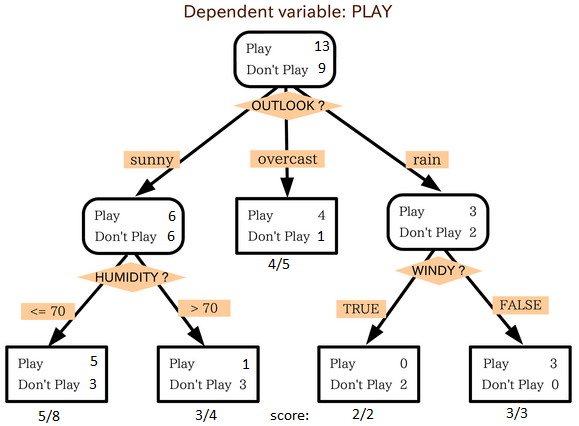Python 代码

1. `#Import Library`
2. `#Import other necessary libraries like pandas, numpy...`
3. `from sklearn import tree`
4. `#Assumed you have, X (predictor) and Y (target) for training data set and x_test(predictor) of test_dataset`
5. `# Create tree object `
6. `model = tree.DecisionTreeClassifier(criterion='gini') # for classification, here you can change the algorithm as gini or entropy (information gain) by default it is gini  `
7. `# model = tree.DecisionTreeRegressor() for regression`
8. `# Train the model using the training sets and check score`
9. `model.fit(X, y)`
10. `model.score(X, y)`
11. `#Predict Output`
12. `predicted= model.predict(x_test)`

R 代码

1. `library(rpart)`
2. `x <- cbind(x_train,y_train)`
3. `# grow tree `
4. `fit <- rpart(y_train ~ ., data = x,method="class")`
5. `summary(fit)`
6. `#Predict Output `
7. `predicted= predict(fit,x_test)`

### 4. 支持向量机（SVM）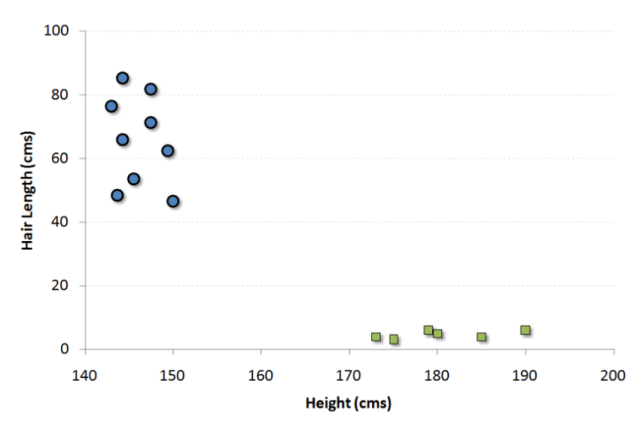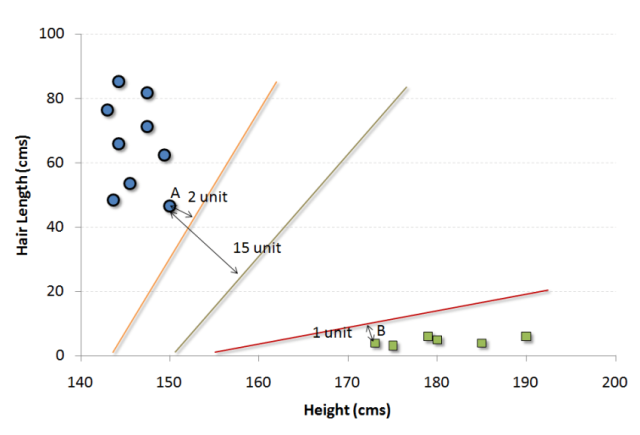#### 我们可以把这个算法想成n维空间里的JezzBall游戏，不过有一些变动：

• 你可以以任何角度画分割线/分割面（经典游戏中只有垂直和水平方向）。
• 现在这个游戏的目的是把不同颜色的小球分到不同空间里。
• 小球是不动的。

Python 代码

1. `#Import Library`
2. `from sklearn import svm`
3. `#Assumed you have, X (predictor) and Y (target) for training data set and x_test(predictor) of test_dataset`
4. `# Create SVM classification object `
5. `model = svm.svc() # there is various option associated with it, this is simple for classification. You can refer link, for mo# re detail.`
6. `# Train the model using the training sets and check score`
7. `model.fit(X, y)`
8. `model.score(X, y)`
9. `#Predict Output`
10. `predicted= model.predict(x_test)`

R 代码

1. `library(e1071)`
2. `x <- cbind(x_train,y_train)`
3. `# Fitting model`
4. `fit <-svm(y_train ~ ., data = x)`
5. `summary(fit)`
6. `#Predict Output `
7. `predicted= predict(fit,x_test)`

### 5. 朴素贝叶斯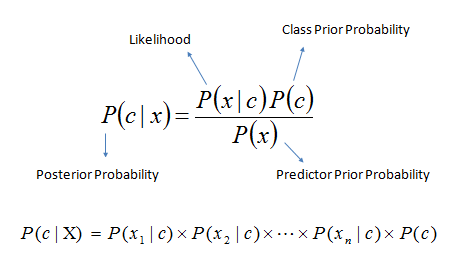• P(c|x)是已知特征x而分类为c的后验概率。
• P(c)是种类c的先验概率。
• P(x|c)是种类c具有特征x的可能性。
• P(x)是特征x的先验概率。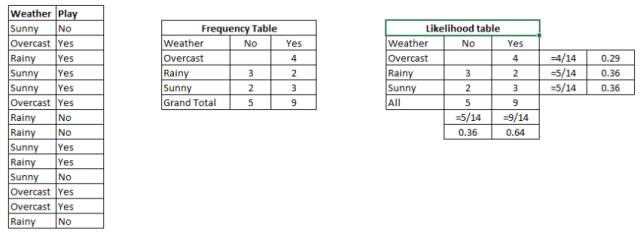Python 代码

1. `#Import Library`
2. `from sklearn.naive_bayes import GaussianNB`
3. `#Assumed you have, X (predictor) and Y (target) for training data set and x_test(predictor) of test_dataset`
4. `# Create SVM classification object model = GaussianNB() # there is other distribution for multinomial classes like Bernoulli Naive Bayes, Refer link`
5. `# Train the model using the training sets and check score`
6. `model.fit(X, y)`
7. `#Predict Output`
8. `predicted= model.predict(x_test)`

R 代码

1. `library(e1071)`
2. `x <- cbind(x_train,y_train)`
3. `# Fitting model`
4. `fit <-naiveBayes(y_train ~ ., data = x)`
5. `summary(fit)`
6. `#Predict Output `
7. `predicted= predict(fit,x_test)`

### 6.KNN（K-邻近算法）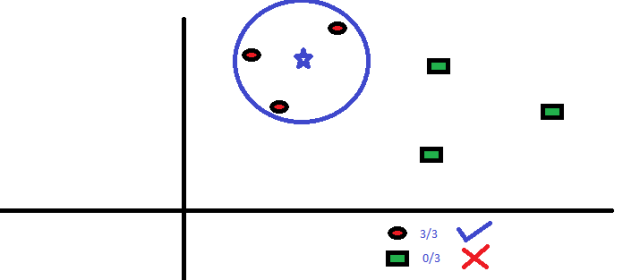KNN在生活中的运用很多。比如，如果你想了解一个不认识的人，你可能就会从这个人的好朋友和圈子中了解他的信息。

• KNN的计算成本很高
• 所有特征应该标准化数量级，否则数量级大的特征在计算距离上会有偏移。
• 在进行KNN前预处理数据，例如去除异常值，噪音等。

Python 代码

1. `#Import Library`
2. `from sklearn.neighbors import KNeighborsClassifier`
3. `#Assumed you have, X (predictor) and Y (target) for training data set and x_test(predictor) of test_dataset`
4. `# Create KNeighbors classifier object model `
5. `KNeighborsClassifier(n_neighbors=6) # default value for n_neighbors is 5`
6. `# Train the model using the training sets and check score`
7. `model.fit(X, y)`
8. `#Predict Output`
9. `predicted= model.predict(x_test)`

R 代码

1. `library(knn)`
2. `x <- cbind(x_train,y_train)`
3. `# Fitting model`
4. `fit <-knn(y_train ~ ., data = x,k=5)`
5. `summary(fit)`
6. `#Predict Output `
7. `predicted= predict(fit,x_test)`

### 7. K均值算法（K-Means）K均值算法如何划分集群：

1. 从每个集群中选取K个数据点作为质心（centroids）。
2. 将每一个数据点与距离自己最近的质心划分在同一集群，即生成K个新集群。
3. 找出新集群的质心，这样就有了新的质心。
4. 重复2和3，直到结果收敛，即不再有新的质心出现。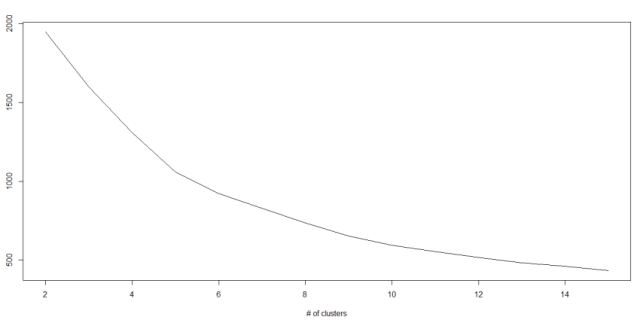Python 代码

1. `#Import Library`
2. `from sklearn.cluster import KMeans`
3. `#Assumed you have, X (attributes) for training data set and x_test(attributes) of test_dataset`
4. `# Create KNeighbors classifier object model `
5. `k_means = KMeans(n_clusters=3, random_state=0)`
6. `# Train the model using the training sets and check score`
7. `model.fit(X)`
8. `#Predict Output`
9. `predicted= model.predict(x_test)`

R 代码

1. `library(cluster)`
2. `fit <- kmeans(X, 3) # 5 cluster solution`

### 8.随机森林

1. 如果训练集中有N种类别，则有重复地随机选取N个样本。这些样本将组成培养决策树的训练集。
2. 如果有M个特征变量，那么选取数m << M，从而在每个节点上随机选取m个特征变量来分割该节点。m在整个森林养成中保持不变。
3. 每个决策树都最大程度上进行分割，没有剪枝。

1. Introduction to Random forest – Simplified
2. Comparing a CART model to Random Forest (Part 1)
3. Comparing a Random Forest to a CART model (Part 2)
4. Tuning the parameters of your Random Forest model

Python 代码

1. `#Import Library`
2. `from sklearn.ensemble import RandomForestClassifier`
3. `#Assumed you have, X (predictor) and Y (target) for training data set and x_test(predictor) of test_dataset`
4. `# Create Random Forest object`
5. `model= RandomForestClassifier()`
6. `# Train the model using the training sets and check score`
7. `model.fit(X, y)`
8. `#Predict Output`
9. `predicted= model.predict(x_test)`

R 代码

1. `library(randomForest)`
2. `x <- cbind(x_train,y_train)`
3. `# Fitting model`
4. `fit <- randomForest(Species ~ ., x,ntree=500)`
5. `summary(fit)`
6. `#Predict Output `
7. `predicted= predict(fit,x_test)`

### 9.降维算法（Dimensionality Reduction Algorithms）

Python 代码

1. `#Import Library`
2. `from sklearn import decomposition`
3. `#Assumed you have training and test data set as train and test`
4. `# Create PCA obeject pca= decomposition.PCA(n_components=k) #default value of k =min(n_sample, n_features)`
5. `# For Factor analysis`
6. `#fa= decomposition.FactorAnalysis()`
7. `# Reduced the dimension of training dataset using PCA`
8. `train_reduced = pca.fit_transform(train)`
9. `#Reduced the dimension of test dataset`
10. `test_reduced = pca.transform(test)`

R 代码

1. `library(stats)`
2. `pca <- princomp(train, cor = TRUE)`
3. `train_reduced  <- predict(pca,train)`
4. `test_reduced  <- predict(pca,test)`

Python 代码

1. `#Import Library`
2. `from sklearn.ensemble import GradientBoostingClassifier`
3. `#Assumed you have, X (predictor) and Y (target) for training data set and x_test(predictor) of test_dataset`
4. `# Create Gradient Boosting Classifier object`
5. `model= GradientBoostingClassifier(n_estimators=100, learning_rate=1.0, max_depth=1, random_state=0)`
6. `# Train the model using the training sets and check score`
7. `model.fit(X, y)`
8. `#Predict Output`
9. `predicted= model.predict(x_test)`

R 代码

1. `library(caret)`
2. `x <- cbind(x_train,y_train)`
3. `# Fitting model`
4. `fitControl <- trainControl( method = "repeatedcv", number = 4, repeats = 4)`
5. `fit <- train(y ~ ., data = x, method = "gbm", trControl = fitControl,verbose = FALSE)`
6. `predicted= predict(fit,x_test,type= "prob")[,2] `